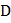# What is the increase in elastic potential energy when the stretching force is increased by 200 kN? a) 238.5 J b) 636.0 J c) 115.5 J d) 79. 5 J

## Question ID - 56053 :- What is the increase in elastic potential energy when the stretching force is increased by 200 kN? a) 238.5 J b) 636.0 J c) 115.5 J d) 79. 5 J

3537

(b)

Initial elastic potential energyLetbe the elongation in the rod when stretching force is  increased by, 200N, Since,OrFinal elastic potential energy isIncrease in elastic potential energy

= 715.5-79.5 = 636.0 J

Next Question :
 The lineintersects the parabolaatand. Normals atandintersect at. Ifis the point at which lineis normal to the parabola, then coordinates ofare a) (4b) (4, 4) c) (d) None of these